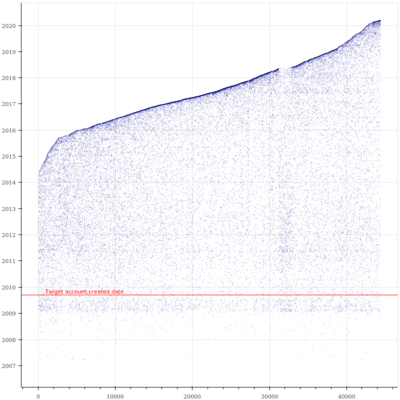# Datetime axis tick intervals query

Linux x64; Python 3.8; bokeh 1.4.0,

This simple code plots an individual twitter account’s followers created_at dates against the order in which the accounts followed.

On the Y axis are datetime objects.

``````def scatter_chart(data, acct_create_date):
""" Make scatter chart """
# data is a tuple of two tuples: datetime objects & sequence no.

p = figure(
plot_width=800,
plot_height=800,
y_axis_type="datetime",
toolbar_location=None,
tools="",
)

acd = Span(
location=acct_create_date,
dimension="width",
line_color="red",
line_dash="solid",
line_width=1.5,
line_alpha=0.4,
)

acd_label = Label(
# text_color=acd.line_color,
text_color="black",
text="    Target account created date",
x=0,
y=acd.location,
text_font="dejavu sans",
text_font_size="8pt",
background_fill_alpha=0.3,
)

p.renderers.extend(
[acd, acd_label,]
)

p.xaxis.formatter = BasicTickFormatter(use_scientific=False)

return p
``````

This gives us

which is fine as far as it goes, but clearly the y_axis tick labels are even-numbered years only, and I’d like to have all the years labelled - first to last inclusive.

I’m guessing I need to add a DatetimeTickFormatter object and reference a list of year strings?

But I’m just an amateur coder & have got stuck.

I’m not tied to 1.4.0, can upgrade if there’s newer features which will assist.

Thanks for any help in advance.

Hi @RedactedCode The location of ticks is controlled by a `Ticker` instance configured on the axis. The default datetime ticker tries to optimize for a “nice” number of ticks, depending on the start/end scale. If you want fixed ticks at a 1-year interval, there is a `YearsTicker` that you can configure on the axis:

``````p.xaxis.ticker = YearsTicker()
``````

(Untested but should be what you need)

1 Like

Thanks, that’s perfect.
I did this:

``````num_years = len({i.strftime("%Y") for i in data})
p.yaxis.ticker = YearsTicker(desired_num_ticks=num_years)
``````

which yields1 Like